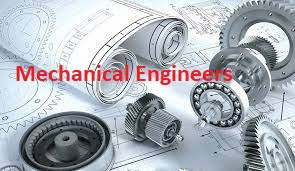### How to calculate the cost of steam???

In power plant, calculation of cost of steam is very vital in commercial point of view. Following are the parameters which affect the cost of steam.

1. Steam pressure
2. Steam temperature
3. GCV of fuel
4. Price of fuel
5. And Boiler efficiency

Following gives you the relation among steam cost & above all parameters & vice versa

• Steam cost increases as the enthalpy or heat content in steam increases and vice versa
• Steam cost increases as the GCV of fuel decreases and vice versa
• Steam cost increases as the fuel price increases & vice versa
• Steam cost increases as the Boiler efficiency decreases & vice versa

Understanding with examples.

1.Calculate the cost of steam per kg, which is been using for Steam turbine having pressure 121 kg/cm2 & temperature 550 deg C.The boiler of efficiency 75% uses coal of GCV 4200 kcal/kg to produce this steam.Cosnsider the price of coal is Rs 5000/MT

Enthalpy of steam at above pressure & temperature H = 830.43 kcal/kg

Boiler efficiency b= 75%

GCV of coal = 4200 kcal/kg

Now, cost of steam = Heat content in steam in kcal/kg  X Fuel price / (GCV of fuel in kcal/kg X Boiler efficiency b)

= 830.43 X 5000 / (4500 X 0.75)

= 1230.26 rupees / MT of steam or Rs 1.23 / kg of steam

2.Calculate the cost of steam per kg, which is been using for chemical process plant having pressure 5 kg/cm2 & temperature 180 deg C.The boiler of efficiency 65% uses biomass of GCV 2800 kcal/kg to produce this steam.Cosnsider the price of biomass is Rs 2400/MT

Enthalpy of steam at above pressure & temperature H = 670 kcal/kg

Boiler efficiency b= 65%

GCV of coal = 2800 kcal/kg

Now, cost of steam = Heat content in steam in kcal/kg X Fuel price / (GCV of fuel in kcal/kg X Boiler efficiency b)

= 670 X 2400 / (2800 X 0.65)

= 883.51 rupees / MT of steam or Rs 0.88 / kg of steam

3. Calculate the cost of saturated steam given to sugar process for juice boiling plant having pressure 1.5 kg/cm2 & temperature 130 deg C.The boiler of efficiency 68% uses bagasse (biomass) of GCV 2200 kcal/kg to produce this steam.Cosnsider the price of biomass is Rs 2500/MT

Enthalpy of steam at above pressure & temperature H = 650 kcal/kg

Boiler efficiency b= 68%

GCV of coal = 2200 kcal/kg

Now, cost of steam = Heat content in steam in kcal/kg X Fuel price / (GCV of fuel in kcal/kg X Boiler efficiency b)

= 650 X 2500 / (2200 X 0.68)

= 1086.22 rupees / MT of steam or Rs 1.086 / kg of steam

Deaeartor & HP heaters steam consumption calculations

### 80-Interview questions & answers for fresher Mechanical Engineers

1.What do you mean by Statics? It is the branch of Engineering Mechanics which deals with the forces & their effects, while acting upo...